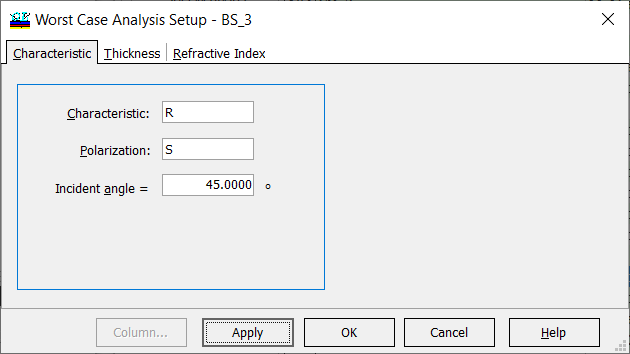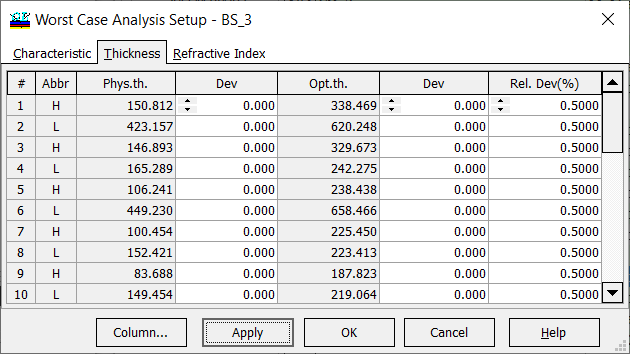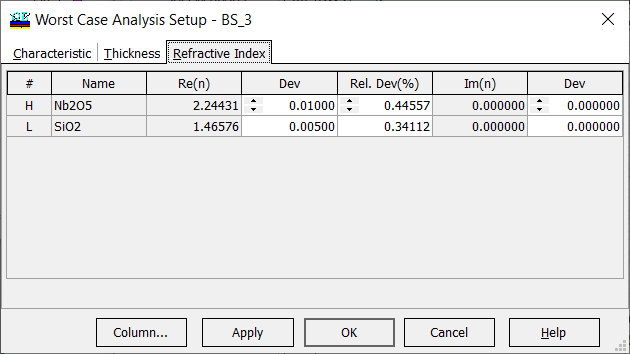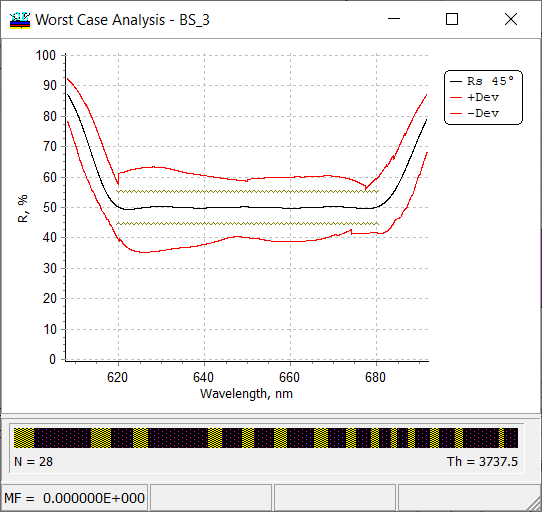### Error analysis: Worst case

 Analysis --> Errors --> Worst case... OptiLayer calculates the largest variations of spectral characteristics provided that layer parameters (thicknesses and refractive indices) can vary within certain limits defined in Worst Case Analysis Setup window. The Worst Case Analysis can be performed only for one spectral characteristic at a time. You should specify the type of this characteristic (Transmittance, Reflectance, etc.), the state of polarization and the angle of incidence prior to calculations (Fig. 1).Fig. 1. Settings for Worst Case Analysis. You can perform the Worst Case Analysis for layer thickness errors and for refractive index errors. In both cases it is necessary to specify maximum allowed parameters deviations in Thickness tab and Refractive Index tab (Figs. 2 and 3).Fig. 2. Example. Maximum allowed errors (0.5%) in layer thicknesses are specified.Fig. 3. Example. Maximum allowed deviations, 0.01 in high-index materials and 0.005 in low-index materials, are specified. The spectral characteristics in the worst case are calculated in the following way. For each wavelength, OptiLayer calculates the spectral characteristic, which are maximally deviates from the nominal characteristic. Then, OptiLayer plots +Dev and -Dev, representing maximal deviations of the disturbed spectral curves from the nominal ones. It is not surprising that +Dev and -Dev are not smooth curves. These curves do not have any physical sense but they give an estimation of the expected deviations.Fig. 4. Example. Worst case analysis of a beamsplitter solution BS_3 with settings shown in Figs. 2 and 3.